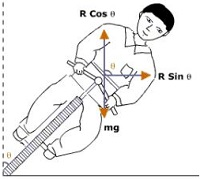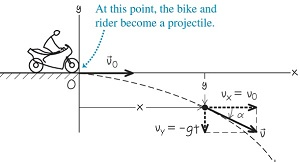# Topic: Motion in A Plane (Test 5)

Topic: Motion in A Plane
Q.1
A cricketer hits a cricket ball from the ground so that it goes directly upwards. If the ball takes, 10 s to return to the ground, determine its maximum height.
A. 132.5 m
B. 112.5 m
C. 152.5 m
D. 122.5 m
Explaination / Solution:

We know that at the maximum height, the velocity of the ball is 0 m/s.

We also know that the ball takes the same time to reach its maximum height as it takes to travel from its maximum height to the initial position, due to time symmetry. The time taken is half the total time.

Therefore, we have the following information for the second (downward) part of the motion of the ball:

t = 5 second; half the total time

g = 9.8 m/s​​​​​​2 downward

s = ?

As we know

s = ut+

Put all the given value,

=> s =

=> s = 122.5 m

Workspace
Report
Q.2
As a ball rises the vertical component of its velocity
A. Can't be determined
B. Increase
C. Decrease
D. Remains constant
Explaination / Solution:

if a ball is thrown upward at an angle? The ball has a vertical and a horizontal component of velocity. The force of gravity is acting on it and its acceleration is in the downward direction. The vertical component of velocity is therefore changing. As the motion and acceleration is in opposite direction so vertical component of velocity decrease.

Workspace
Report
Q.3
The reason why cyclists bank when taking a sharp turn is
A. to supply the sidewise (centripetal) acceleration required to make the direction change
B. cyclists enjoy turning to one side and so bank
C. to supply the acceleration required to move fast
D. to decelerate at the turns as turns are dangerous
Explaination / Solution:

In order to take a safe turn, the cyclist has to bend a little from his vertical position. In this case, a component of the reaction provides the required centripetal force.

If q is angle made by the cyclist with the vertical then

#####Dividing (2) by (1), we get

=>

In actual practice, the value of q is slightly less because the force of friction also contributes towards the centripetal force.

Workspace
Report
Q.4
A motorcycle stunt rider rides off the edge of a cliff. Just at the edge his velocity is horizontal, with magnitude 9.0 m/s. Find the magnitude of the motorcycle’s position vector after 0.50s it leaves the edge of the cliff.
A. 3.5 m
B. 4.7 m
C. 4.3 m
D. 5.2 m
Explaination / Solution:

The motorcycle’s x- and  y-coordinates at t=0.50 s arex = vox t=  =4.5 m

y=  =  -1.2m

The negative value of y shows that the motorcycle is below its starting point.

The motorcycle’s distance from the origin at t=0.50 s is r= =4.7 m

Workspace
Report
Q.5

A sports car has a “lateral acceleration” of 0.96g = 9.4 m . This is the maximum centripetal acceleration the car can sustain without skidding out of a curved path. If the car is traveling at a constant 40 m/s on level ground, what is the radius R of the tightest unbanked curve it can negotiate?

A. minimum radius = 180 m
B. minimum radius = 160 m
C. minimum radius = 170 m
D. minimum radius = 175 m
Explaination / Solution:

The car is in uniform circular motion because it’s moving at a constant speed along a curve that is a segment of a circle. Hence we know

R==170 m

This is the minimum turning radius because arad is the maximum centripetal acceleration.

Workspace
Report
Q.6
Passengers on a carnival ride move at constant speed in a horizontal circle of radius 5.0 m, making a complete circle in 4.0 s. What is their acceleration?
A. 16 m
B. 12 m
C. 14 m
D. 15 m
Explaination / Solution:

The speed is constant, so this is uniform circular motion. We are given the radius R=5.0 m and the period T=4.0 s, so we can  calculate the acceleration directly using equation

== 12m/s​​​​​​2

Workspace
Report
Q.7
A projectile is fired a velocity of 150 meters per second at an angle of 30 degrees with the horizontal. What is the magnitude of the vertical component of the velocity at the time the projectile is fired?
A. 75 m/s
B. 150 m/s
C. 225 m/s
D. 130 m/s
Explaination / Solution:

Initial velocity u = 150 m/s

Angle

Vertical component is given by

= 75 m/s

Workspace
Report
Q.8

At a distance L=400m from the traffic lights the brakes are applied to a locomotive moving at a velocity v=54km/hr. Determine the position of locomotive relative to the traffic lights 1 minute after the application of the brakes if its acceleration is -0.3m/s​​​​​​2.

A. 15 m
B. 25 m
C. 30 m
D. 40 m
Explaination / Solution:

Initial velocity u = 54 km/h = 15 m/s

Let Final velocity v = 0

Acceleration a = -0.3 m/s​​​​​​2

Time taken to stop = t

Using

=>

=> t = 50 sec

It means it has been stopped before 1 minutes.

So distance covered in 1 minute

=>

=> s = 375 m

Position of locomotive relative to the traffic lights= 400-375 = 25 m

Workspace
Report
Q.9
A. translating the two vectors
C. adding the angles of the vectors
D. adding the magnitudes of the vectors
Explaination / Solution:

Parallelogram law of vector addition is,If two vectors are considered to be the adjacent sides of a Parallelogram, then the resultant of two vectors is given by the vector which is a diagonal passing through the point of contact of two vectors.

Workspace
Report
Q.10